Download Presentation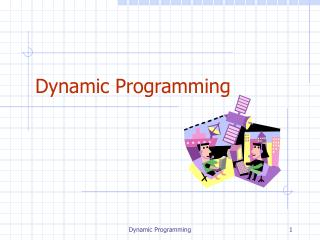Dynamic Programming

# Dynamic Programming - PowerPoint PPT Presentation

Dynamic Programming. Outline and Reading. Matrix Chain-Product ( §5.3.1 ) The General Technique ( §5.3.2 ) 0-1 Knapsack Problem ( §5.3.3 ). Dynamic Programming is a general algorithm design paradigm. Rather than give the general structure, let us first give a motivating example:I am the owner, or an agent authorized to act on behalf of the owner, of the copyrighted work described.
Download Presentation## Dynamic Programming

An Image/Link below is provided (as is) to download presentation

Download Policy: Content on the Website is provided to you AS IS for your information and personal use and may not be sold / licensed / shared on other websites without getting consent from its author.While downloading, if for some reason you are not able to download a presentation, the publisher may have deleted the file from their server.

- - - - - - - - - - - - - - - - - - - - - - - - - - E N D - - - - - - - - - - - - - - - - - - - - - - - - - -
Presentation Transcript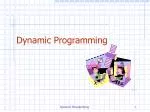### Dynamic Programming

Dynamic Programming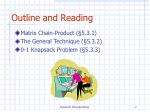• Matrix Chain-Product (§5.3.1)
• The General Technique (§5.3.2)
• 0-1 Knapsack Problem (§5.3.3)

Dynamic Programming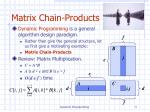Dynamic Programming is a general algorithm design paradigm.

Rather than give the general structure, let us first give a motivating example:

Matrix Chain-Products

Review: Matrix Multiplication.

C = A*B

Aisd × eandBise × f

O(def)time

f

B

j

e

e

A

C

d

i

i,j

d

f

Matrix Chain-Products

Dynamic Programming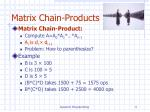Matrix Chain-Product:

Compute A=A0*A1*…*An-1

Ai is di × di+1

Problem: How to parenthesize?

Example

B is 3 × 100

C is 100 × 5

D is 5 × 5

(B*C)*D takes 1500 + 75 = 1575 ops

B*(C*D) takes 1500 + 2500 = 4000 ops

Matrix Chain-Products

Dynamic Programming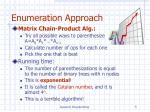Matrix Chain-Product Alg.:

Try all possible ways to parenthesize A=A0*A1*…*An-1

Calculate number of ops for each one

Pick the one that is best

Running time:

The number of parenthesizations is equal to the number of binary trees with n nodes

This is exponential!

It is called the Catalan number, and it is almost 4n.

This is a terrible algorithm!

Enumeration Approach

Dynamic Programming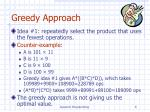Counter-example:

A is 101 × 11

B is 11 × 9

C is 9 × 100

D is 100 × 99

Greedy idea #1 gives A*((B*C)*D)), which takes 109989+9900+108900=228789 ops

(A*B)*(C*D) takes 9999+89991+89100=189090 ops

The greedy approach is not giving us the optimal value.

Greedy Approach

Dynamic Programming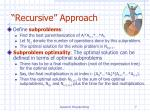“Recursive” Approach
• Define subproblems:
• Find the best parenthesization of Ai*Ai+1*…*Aj.
• Let Ni,j denote the number of operations done by this subproblem.
• The optimal solution for the whole problem is N0,n-1.
• Subproblem optimality: The optimal solution can be defined in terms of optimal subproblems
• There has to be a final multiplication (root of the expression tree) for the optimal solution.
• Say, the final multiplication is at index i: (A0*…*Ai)*(Ai+1*…*An-1).
• Then the optimal solution N0,n-1 is the sum of two optimal subproblems, N0,i and Ni+1,n-1 plus the time for the last multiplication.

Dynamic Programming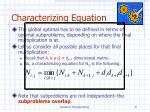Characterizing Equation
• The global optimal has to be defined in terms of optimal subproblems, depending on where the final multiplication is at.
• Let us consider all possible places for that final multiplication:
• Recall that Ai is a di× di+1 dimensional matrix.
• So, a characterizing equation for Ni,j is the following:
• Note that subproblems are not independent–the subproblems overlap.

Dynamic Programming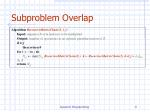Subproblem Overlap

AlgorithmRecursiveMatrixChain(S, i, j):

Input:sequence S of n matrices to be multiplied

Output:number of operations in an optimalparenthesization of S

if i=j

then return 0

forkito jdo

Ni, jmin{Ni,j, RecursiveMatrixChain(S, i ,k)+RecursiveMatrixChain(S, k+1,j)+ di dk+1dj+1}

returnNi,j

Dynamic Programming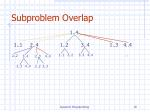Subproblem Overlap

1..4

1..1

2..4

1..2

3..4

1..3

4..4

1..1

2..2

3..3

4..4

...

2..2

3..4

2..3

4..4

3..3

4..4

2..2

3..3

Dynamic ProgrammingDynamic Programming Algorithm

AlgorithmmatrixChain(S):

Input:sequence S of n matrices to be multiplied

Output:number of operations in an optimal parenthesization of S

fori 1 to n - 1do

Ni,i0

forb1to n - 1do

{ b =j - iis the length of the problem }

fori0to n - b -1do

ji + b

Ni,j+

forkito j - 1do

Ni,jmin{Ni,j, Ni,k + Nk+1,j + di dk+1dj+1}

returnN0,n-1

• Since subproblems overlap, we don’t use recursion.
• Instead, we construct optimal subproblems “bottom-up.”
• Ni,i’s are easy, so start with them
• Then do problems of “length” 2,3,… subproblems, and so on.
• Running time: O(n3)

Dynamic ProgrammingDynamic Programming Algorithm Visualization

• The bottom-up construction fills in the N array by diagonals
• Ni,j gets values from previous entries in i-th row and j-th column
• Filling in each entry in the N table takes O(n) time.
• Total run time: O(n3)
• Getting actual parenthesization can be done by remembering “k” for each N entry

N

0

1

2

i

j

n-1

0

1

i

j

n-1

Dynamic ProgrammingDynamic Programming Algorithm Visualization
• A0: 30 X 35; A1: 35 X15; A2: 15X5;

A3: 5X10; A4: 10X20; A5: 20 X 25

Dynamic ProgrammingDynamic Programming Algorithm

AlgorithmmatrixChain(S):

Input:sequence S of n matrices to be multiplied

Output:number of operations in an optimal parenthesization of S

fori 1 to n - 1do

Ni,i0; Tiii;

forb1to n - 1do

{ b =j - iis the length of the problem }

fori0to n - b -1do

ji + b

Ni,j+; Tiji;

forkito j - 1do

If (Ni,j>Ni,k + Nk+1,j + di dk+1dj+1)

Ti,jk

Ni,jmin{Ni,j, Ni,k + Nk+1,j + di dk+1dj+1}

returnN0,n-1

• Since subproblems overlap, we don’t use recursion.
• Instead, we construct optimal subproblems “bottom-up.”
• Ni,i’s are easy, so start with them
• Then do problems of “length” 2,3,… subproblems, and so on.
• Running time: O(n3)

Dynamic ProgrammingDynamic Programming Algorithm Visualization

(A0*(A1*A2))*((A3*A4)*A5)

Dynamic ProgrammingThe General Dynamic Programming Technique
• Applies to a problem that at first seems to require a lot of time (possibly exponential), provided we have:
• Subproblem optimality: the global optimum value can be defined in terms of optimal subproblems
• Subproblem overlap: the subproblems are not independent, but instead they overlap (hence, should be constructed bottom-up).

Dynamic ProgrammingThe 0/1 Knapsack Problem
• Given: A set S of n items, with each item i having
• wi - a positive weight
• bi - a positive benefit
• Goal: Choose items with maximum total benefit but with weight at most W.
• If we are not allowed to take fractional amounts, then this is the 0/1 knapsack problem.
• In this case, we let Tdenote the set of items we take
• Objective: maximize
• Constraint:

Dynamic ProgrammingExample
• Given: A set S of n items, with each item i having
• bi - a positive “benefit”
• wi - a positive “weight”
• Goal: Choose items with maximum total benefit but with weight at most W.

“knapsack”

Items:

box of width 9 in

1

2

3

4

5

• Solution:
• item 5 (\$80, 2 in)
• item 3 (\$6, 2in)
• item 1 (\$20, 4in)

Weight:

4 in

2 in

2 in

6 in

2 in

Benefit:

\$20

\$3

\$6

\$25

\$80

Dynamic ProgrammingA 0/1 Knapsack Algorithm, First Attempt
• Sk: Set of items numbered 1 to k.
• Define B[k] = best selection from Sk.
• Problem: does not have subproblem optimality:
• Consider set S={(3,2),(5,4),(8,5),(4,3),(10,9)} of(benefit, weight) pairs and total weight W = 20

Best for S4:

Best for S5:

Dynamic ProgrammingA 0/1 Knapsack Algorithm, Second Attempt
• Sk: Set of items numbered 1 to k.
• Define B[k,w] to be the best selection from Sk with weight at most w
• Good news: this does have subproblem optimality.
• I.e., the best subset of Sk with weight at most w is either
• the best subset of Sk-1 with weight at most w or
• the best subset of Sk-1 with weight at most w-wk plus item k

Dynamic Programming0/1 Knapsack Algorithm
• Consider set S={(1,1),(2,2),(4,3),(2,2),(5,5)} of (benefit, weight) pairs and total weight W = 10

Dynamic Programming0/1 Knapsack Algorithm
• Trace back to find the items picked

Dynamic Programming0/1 Knapsack Algorithm
• Each diagonal arrow corresponds to adding one item into the bag
• Pick items 2,3,5
• {(2,2),(4,3),(5,5)} are what you will take away

Dynamic Programming0/1 Knapsack Algorithm
• Recall the definition of B[k,w]
• Since B[k,w] is defined in terms of B[k-1,*], we can use two arrays of instead of a matrix
• Running time: O(nW).
• Not a polynomial-time algorithm since W may be large
• This is a pseudo-polynomial time algorithm

Algorithm01Knapsack(S,W):

Input:set S of n items with benefit biand weight wi; maximum weight W

Output:benefit of best subset of S with weight at most W

letAandBbe arrays of lengthW + 1

forw 0to W do

B[w]0

fork 1 to n do

copy arrayBinto arrayA

forwwkto Wdo

ifA[w-wk] + bk > A[w]then

B[w]A[w-wk] + bk

returnB[W]

Dynamic ProgrammingLongest Common Subsequence
• Given two strings, find a longest subsequence that they share
• substring vs. subsequence of a string
• Substring: the characters in a substring of S must occur contiguously in S
• Subsequence: the characters can be interspersed with gaps.
• Consider ababc and abdcb

alignment 1

ababc.

abd.cb

the longest common subsequence is ab..c with length 3

alignment 2

aba.bc

abdcb.

the longest common subsequence is ab..b with length 3

Dynamic ProgrammingLongest Common Subsequence

Let’s give a score M an alignment in this way,

M=sum s(xi,yi), where xi is the i character in the first aligned sequence

yi is the i character in the second aligned sequence

s(xi,yi)= 1 if xi= yi

s(xi,yi)= 0 if xi≠yi or any of them is a gap

The score for alignment:

ababc.

abd.cb

M=s(a,a)+s(b,b)+s(a,d)+s(b,.)+s(c,c)+s(.,b)=3

To find the longest common subsequence between sequences S1 and S2 is to find the alignment that maximizes score M.

Dynamic ProgrammingLongest Common Subsequence
• Subproblem optimality

Consider two sequences

Let the optimal alignment be

x1x2x3…xn-1xn

y1y2y3…yn-1yn

There are three possible cases

for the last pair (xn,yn):

S1: a1a2a3…ai

S2: b1b2b3…bj

Dynamic ProgrammingLongest Common Subsequence

There are three cases for (xn,yn) pair:

Mi,j = MAX {Mi-1, j-1 + S (ai,bj) (match/mismatch)

Mi,j-1 + 0 (gap in sequence #1)

Mi-1,j + 0 (gap in sequence #2) }

Mi,j is the score for optimal alignment between strings a[1…i] (substring of a from index 1 to i) and b[1…j]

S1: a1a2a3…ai

S2: b1b2b3…bj

x1x2x3…xn-1xn

y1y2y3…yn-1yn

Dynamic ProgrammingLongest Common Subsequence

Mi,j = MAX {

Mi-1, j-1 + S(ai,bj)

Mi,j-1 + 0

Mi-1,j + 0

}

Examples:

G A A T T C A G T T A (sequence #1)

G G A T C G A (sequence #2)

s(ai,bj)= 1 if ai=bj

s(ai,bj)= 0 if ai≠bjor any of them is a gap

Dynamic ProgrammingLongest Common Subsequence

Fill the score matrix M and trace back table B

M1,1 = MAX[M0,0 + 1, M1, 0 + 0, M0,1 + 0] = MAX [1, 0, 0] = 1

Score matrix M

Trace back table B

Dynamic ProgrammingLongest Common Subsequence

Score matrix M

Trace back table B

M7,11=6 (lower right corner of Score matrix)

This tells us that the best alignment has a score of 6

What is the best alignment?

Dynamic ProgrammingLongest Common Subsequence

We need to use trace back table to find out the best alignment,

which has a score of 6

• Find the path from lower right
• corner to upper left corner

Dynamic ProgrammingLongest Common Subsequence

(2) At the same time, write down the alignment backward

S1

:Take one character from each sequence

:Take one character from sequence S1 (columns)

S2

:Take one character from sequence S2 (rows)

Dynamic ProgrammingLongest Common Subsequence

:Take one character from each sequence

:Take one character from sequence S1 (columns)

:Take one character from sequence S2 (rows)

Dynamic ProgrammingLongest Common Subsequence

Thus, the optimal alignment is

The longest common subsequence is

G.A.T.C.G..A

There might be multiple longest common subsequences (LCSs)

between two given sequences.

These LCSs have the same number of characters (not include gaps)

Dynamic ProgrammingLongest Common Subsequence

Algorithm LCS(string A, string B) {

Input strings A and B

Output the longest common subsequence of A and B

M: Score Matrix

B: trace back table (use letter a, b, c for )

n=A.length()

m=B.length()

// fill in M and B

for (i=0;i<m+1;i++)

for (j=0;j<n+1;j++)

if (i==0) || (j==0)

then M(i,j)=0;

else if (A[i]==B[j])

M(i,j)=max {M[i-1,j-1]+1, M[i-1,j], M[i,j-1]}

{update the entry in trace table B}

else

M(i,j)=max {M[i-1,j-1], M[i-1,j], M[i,j-1]}

{update the entry in trace table B}

then use trace back table B to print out the optimal alignment

Dynamic Programming